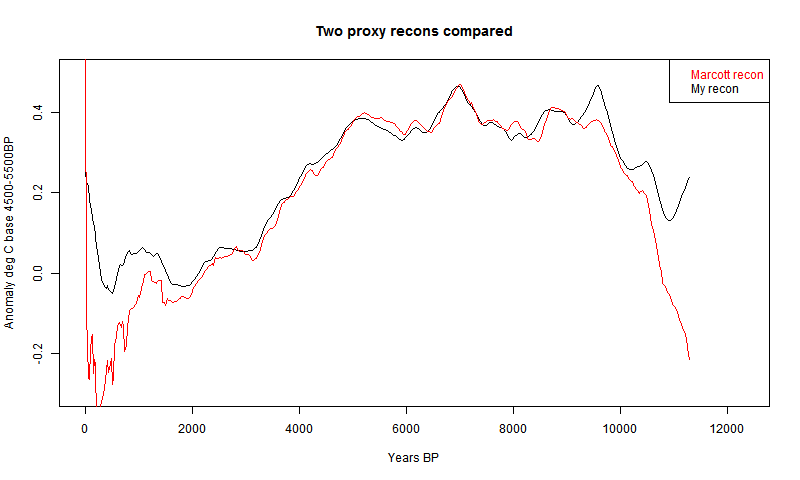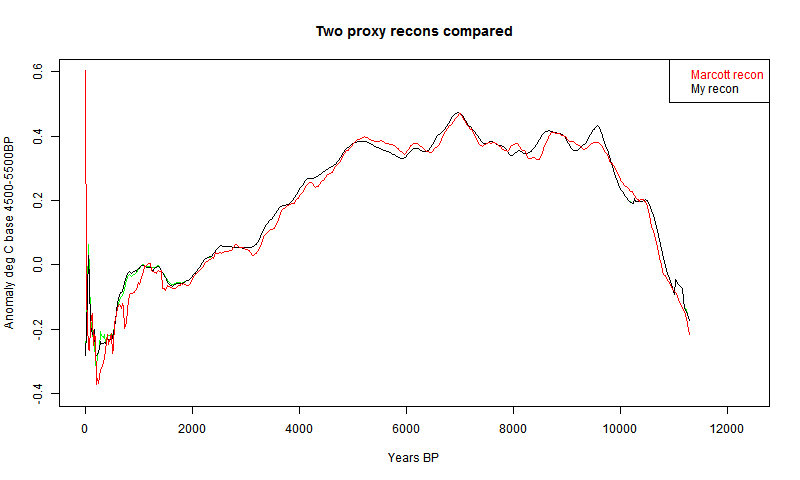## Friday, April 26, 2013

### ## Emulating Marcott et al

Update - error found and fixed. Agreement of 2nd recon below is now good.

I have now posted three "elementary" emulations of Marcott et al. Each, in its own way, was a year by year average (here, here, and here). As such, it missed the distinctive feature of Marcott et al, which was the smoothing produced by Mante Carlo modelling of the dating uncertainty. So here I am trying to grapple with that.

A story - I used to share a computer system with a group of statisticians. It worked well (for me) for a while - they used Genstat, GLIM etc - no big computing. But then some developed an enthusiasm for Monte Carlo modelling. I've had an aversion to it since. So here, I try to use exact probability integrals instead. There are clearly big benefits in computing time. Marcott et al had to scale their MC back to 1000 repetitions for the Science article, and I'm sure they have big computers. My program takes a few seconds.

A reconstruction is basically a function F(d,t,e) where d is data, t time, and e a vector of random variables. MC modelling means averaging over a thousand or so realizations of e from its joint distribution. But this can also be written as a probability integral:
R(t)=∫F(d,t,e) dΦ(e)
where Φ is the cumulative distribution function.

MC modelling means basically evaluating that integral by the Monte Carlo method. For high dimensionality this is a good alternative, but if the variables separate to give integrals in one or few variables, MC is very inefficient.

The aim here is to separate the stochastic variables and integrate analytically in single variables. The scope is just to generate the recon - the CI's will wait for another day

#### Variables, date uncertainty and basis functions

The data consists of a set of proxies calibrated to temperature. There is uncertainty associated with the temperature calibration, and of course there is general variability which will ensure that no reconstruction is exactly fitted; this is modelled as residual noise. For the purpose of calculating the mean recon, these added stochastic variables will in a Monte Carlo converge to their mean, which is zero. So they needn't be included.

The variable that is important is the age (date) uncertainty. This arises from various things - the mixing of material of different ages that may have occurred; reservoir times, meaning that the proxy material may not have actually reached the sampling site for some years, and uncertainty in the actual dating, which is mostly based on Carbon 14.

In a post a few days ago, I described how an interpolated function can be described as the product of data values with triangle basis functions. That will be used here. The date uncertainty becomes a stochastic translation of the basis functions.

#### Random variables

As mentioned above, if the analytic integral approach is to succeed, it will be desirable to partition the variation into items affected by a single (ideally) independent random variable. In fact, for dating an obvious candidate would be the C14 point uncertainty, which affects the data ages through the age model construction. I could not see how to actually do this, and Marcott et al give individual age uncertainties to the data points, as well as C14 uncertainties. Furthermore the data uncertainties are much higher than they would have inherited by interpolation from the C14 points, so I assume other sources of uncertaintyt are included. Still one would not expect the data points to vary independently, but no other information is given. I came to believe that Marcott et al did vary them independently, and in terms of the amount of smoothing that results, it may not matter.

#### Integrals

I treated the basis functions in the finite element style described in the previous post. That is, I summed them for each proxy by intervals, with two half-triangles at a time. Let a data value f0 is associated with a triangle basis function B01(t) on the range t0 to t1 (where t1 is the next data point), and B01(t)=1-(t-t0)/dt, dt=(t1-t0). If the date uncertainty of t0 is s0, then the probability integral expressing the expected value of product at time t is
∫ f0*B0(1+(t0+s0*e-t)/dt) f(e) de
where f(e) is the unit normal probability density function (Gaussian). The limits of the integral are determined by B being between 1 and 0.

There is a wrinkle here - both t0 and t1 have error, and you might expect that to be reflected in the difference dt. If the error in t1 were independent, then t1 might easily become less than t0 - ie data points would get out of order. However, with age model uncertainty that won't happen; the interpolated data ages will just slide along in parallel, and dt will not vary much at all.

So I thought that a much more reasonable assumption is that B can translate, but not contract.

As mentioned, I sum two parts of the basis on each interval, to make up a linear interpolate. The algebra all works if t0 and t1 are interchanged.

#### Evaluation

The probability integral is linear in e, and easily worked out:
$\frac{t1-t}{dt} (\Phi(\frac{t1-t}{\sigma})-\Phi(\frac{t0-t}{\sigma})) +\frac{\sigma}{dt}(\phi(\frac{t1-t}{\sigma})-\phi(\frac{t0-t}{\sigma}))$
where Φ is the cumulative normal.

The time values t are Marcott's 20 year intervals, going back from 1940 (10BP) to 11290BP. So this process of smoothed basis functions effects the interpolation on to those points, on which the different proxies are then aggregated.

#### End conditions

The operation of this integral process is like gaussian smoothing, except that s changes from point to point. So some notion is needed of values beyond the range of proxy data, if it does not extend the full range. This is indeed the bugbear of the analysis.

My first inclination was just to extend the first and last values, although I did not let them go to negative ages BP. That worked fairly well, as I shall show.

#### First reconstruction

My first recon was much as described here, but with the date process above added. The 73 proxies were located in 5x5° lat/lon cells. Time data at the 20-year intervals was calculated for each proxy. Then cell averages were computed, and then an area-weighted mean (allowing for latitude variation of cell area). At each time point, of course, only the proxies with data are included in the sum, and the weights are adjusted at each 20yr point to allow for missing proxies.It's mostly quite a good fit, and clearly has about the right degree of smoothing. But it diverges somewhat in early Holocene and much more close to present. This is likely to be due to proxies coming to data endpoints, so I thought a more thorough treatment was required.

#### Better end treatment

So I looked more carefully at what would happen in a Monte Carlo near data endpoints. At the start, the first age point moves back and forth, so that some of the 20-yr points are sometimes in and sometimes out of the data range. That implies a change in the cross-proxy weighting. When that proxy is considered missing, it's weight goes to zero and other weights rise (to add to 1).

That's bad news for my method, which tries to keep the effect of the random variables isolated to a single proxy data point. This would spread its effect right across.

However, there is another way of allowing for missing data in an average which I find useful. You get exactly the same result if you don't change the weights but replacing the missing value with the (weighted) average of the remaining points. Then the weights don't need to change.

The problem is, we don't have that value yet; we're doing the proxies one by one. The solution is, iteration. The ending proxy is just one of at least fifty. It doesn't make that much difference to the aggregate. If we substitute the numbers from the first recon, say, then the second time around we'll have a much more accurate value. A third iteration is not needed.

There is a subtlety here where there are several in a cell. Then when one goes missing, it should be replaced by the cell average. It is only when the proxy was alone in a cell, that it should be replaced by the recon average when it goes missing. The test is always, what value should it have so that the aggregate would be the same as if the remainder were averaged without it.

#### Second recon

So I did that. That is, I in effect padded with the recon average from a previous iteration, or the cell average if appropriate. The result was thisI had previously made an error with the cell area weighting, which affected this plot. Now fixed.
It's much better at the start. In fact, it even almost reproduces Marcott's non-robust 1940 spike (well, after fixing a start problem, it's now a 1900 spike followed by a dive). I fixed a problem at the early end, so that I now use data beyond the end where available in the integral, as Marcott seems to have done with Monte Carlo. So agreement is good there too..

1.I wonder if there is an issue with a slight difference in grid definition such that, for example, a proxy close to a grid edge could be on its own or averaged with another proxy with only a small shift in grid setup assumptions. This would be particularly relevant for some proxies in the North Atlantic which contain such large downward trends that they can significantly impact the global average on their own.

I wonder if you could test by varying offsets for 5º grid spacing to define an uncertainty range, e.g. 0 - 5º then 1 - 6º then 2 - 7º etc.

1.Paul,
I think varying grid size would be another thing. For 5x5, there are only 11 cells with more than one proxy, and for at least three of those, the proxies are from the same core.

I'd be surprised if Marcott et al didn't use the same cells I did. They'd have to start latitude at -90, and it would be odd to use a different convention for longitude.